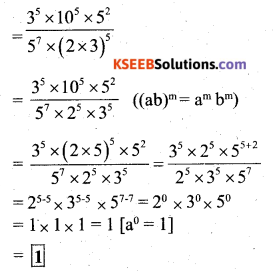# KSEEB Solutions for Class 7 Maths Chapter 13 Exponents and Powers Ex 13.2

Students can Download Chapter 13 Exponents and Powers Ex 13.2, Question and Answers, Notes Pdf, KSEEB Solutions for Class 7 Maths, Karnataka State Board Solutions help you to revise complete Syllabus and score more marks in your examinations.

## Karnataka State Syllabus Class 7 Maths Chapter 13 Exponents and Powers Ex 13.2

Question 1.
Using laws of exponents, simplify and write the answer in exponential form :
(i) 32 × 34 × 38
(ii) 615 ÷ 610
(iii) a3 × a2
(iv) 7x × 72
(v) (52)3 ÷ 52
(vi) 25 × 55
(vii) (a4 × b4)
(viii) (34)3
(ix) (220 ÷ 215) × 23
(x) 8t ÷ 82
Solution:
i) 32 × 34 × 38
= 32 + 4 + 8 = 314 [∵ am × an = am + n]

ii) 615 ÷ 610
= 615 – 10 = 65 [∵ am ÷ an = am – n]

iii) a3 × a2
= a3 + 2 = a5 [∵ am × an = am + n]

iv) 7x × 72
= 7x + 2 [∵ am × an = am + n]

v) (52)3 ÷ 53
= 52 × 3 ÷ 53 = 56 – 3 [(am)n = amn
∵ am ÷ an = am – n]

vi) 25 × 55
= (2 × 5)5 = 105 [∵ am × bm = (ab)m]

vii) a4 × b4
= a4 × b4 = (ab)4 [∵ am × bm = (ab)m]

viii) (34)3
= 34 × 3 = 312 [(am)n = amn]

ix) (220 ÷ 215)
(220 ÷ 215) × 23 = 220 – 15 × 23 [am ÷ an = am – n]
= 25 × 23
= 25 + 3 = 28 [am × an = am + n]

x) 8t ÷ 82
= 8t – 2 [∵ am ÷ an = am – n]Question 2.
Simplify and express each of the following in exponential form :
i)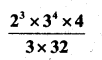Solution: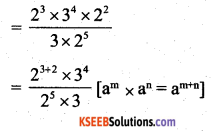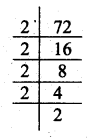ii) ((52)3 × 54) ÷ 57
= (52 × 3 × 54) ÷ 57 ((am)n = amn)
= 56 × 54 ÷ 57
= 56 + 4 ÷ 57 = 510 – 7 = 53

iii) 254 ÷ 53
= (52)4 ÷ 53 = 52 × 4 ÷ 53
= 58 ÷ 53 = 58 – 3 = 55 [am ÷ an = am – n

iv)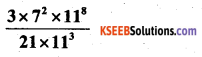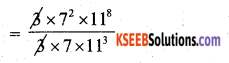= 72 – 1 × 118 – 3 [am ÷ an = am – n]
= 71 × 115 = 7 × 115

vi) 20 + 30 + 40
= 1 + 1 + 1 =  [a0 = 1]

vii) 20 × 30 × 40
= 1 × 1 × 1 = 1

viii) (30 + 20) × 50
= (1 + 1) × 1 = 2 × 1 = 2

ix)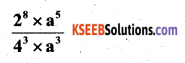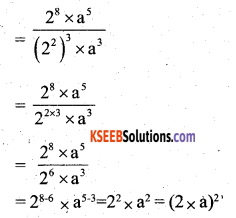= (2a)2

x)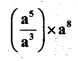= a 5 – 3 × a8
= a2 × a8 a2 + 8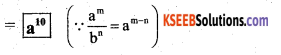xi)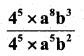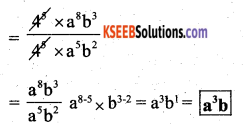xii) (23 × 2)2
= (23 × 21)2
= (24)2
= 24 × 2 = 28

Question 3.
i) 10 × 1011 = 10011
L.H.S = 10 × 1011 = 101 + 11 = 1012 RHS
10011 = (10 × 10)11
= (102)11
= 1o2 × 11 = 1022
∴ 1012 ≠ 1022
So 10 × 1011 ≠ 10011 ∴ It is false.

ii) 23 > 52
= 2 × 2 × 2 > 5 × 5
= 8 > 25
∴ 23 > 52 ∴ It is false.

iii) 23 × 32 = 65
= 2 × 2 × 2 × 3 × 3
= 6 × 6 × 6 × 6 × 6
= 72 = 7776
∴ 23 × 32 ≠ 65 ∴ It is false.

iv) 30 = (1000)0
1 = 1
∴ 30 = (1000)0 ∴ It is trueQuestion 4.
Express each of the following as a product of prime factors only in exponential form :

i) 108 x 192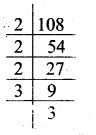= 2 × 2 × 3 × 3 × 3 2 × 2 × 2 × 2 × 2 × 2 × 3
= 2 × 2 × 2 × 2 × 2 × 2 × 2 × 2 × 3 × 3 × 3 × 3
= 28 × 34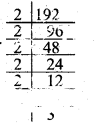It is the product of prime factor

ii) 270
270 = 2 × 3 × 3 × 3 × 5
= 2 × 33 × 5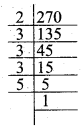It is required prime factor.

iii) 729 × 64
729 = 3 × 3 × 3 × 3 × 3 × 3
64 = 2 × 2 × 2 × 2 × 2 × 2
∴ 729 × 64 = 36 × 26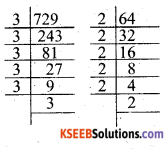It is the required prime factor.

iv) 768
768 = 2 × 2 × 2 × 2 × 2 × 2 × 2 × 2 × 3
= 28 × 31 or = 28 × 3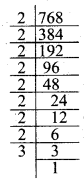It is a required prime factor.

Question 5.
Simplify :
i)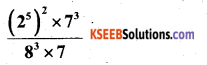Solution: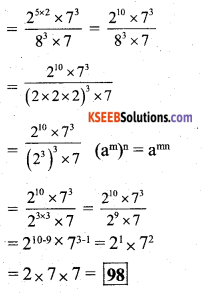ii)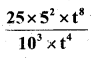Solution: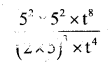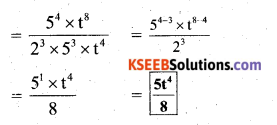iii)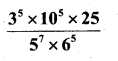Solution: